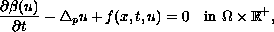Electron. J. Diff. Eqns., Vol. 2003(2003), No. 113, pp. 1-14.

### Doubly nonlinear parabolic equations related to the p-Laplacian operator: Semi-discretization Fatiha Benzekri & Abderrahmane El Hachimi

Abstract:
We study the doubly nonlinear parabolic equationwith Dirichlet boundary conditions and initial data. We investigate a time-discretization of the continuous problem by the Euler forward scheme. In addition to proving existence, uniqueness and stability questions, we study the long time behavior of the solution to the discrete problem. We prove the existence of a global attractor, and obtain its regularity under additional conditions.

Submitted April 16, 2003. Published November 11, 2003.
Math Subject Classifications: 35K15, 35K60, 35J60.
Key Words: P-Laplacian, nonlinear parabolic equations, semi-discretization, discrete dynamical system, attractor.

Show me the PDF file (240K), TEX file, and other files for this article.Fatiha Benzekri UFR Mathematiques Appliquees et Industrielles Faculte des sciences B. P. 20, El Jadida, Maroc email: benzekri@ucd.ac.ma Abderrahmane El Hachimi UFR Mathematiques Appliquees et Industrielles Faculte des sciences B. P. 20, El Jadida, Maroc email: elhachimi@ucd.ac.ma